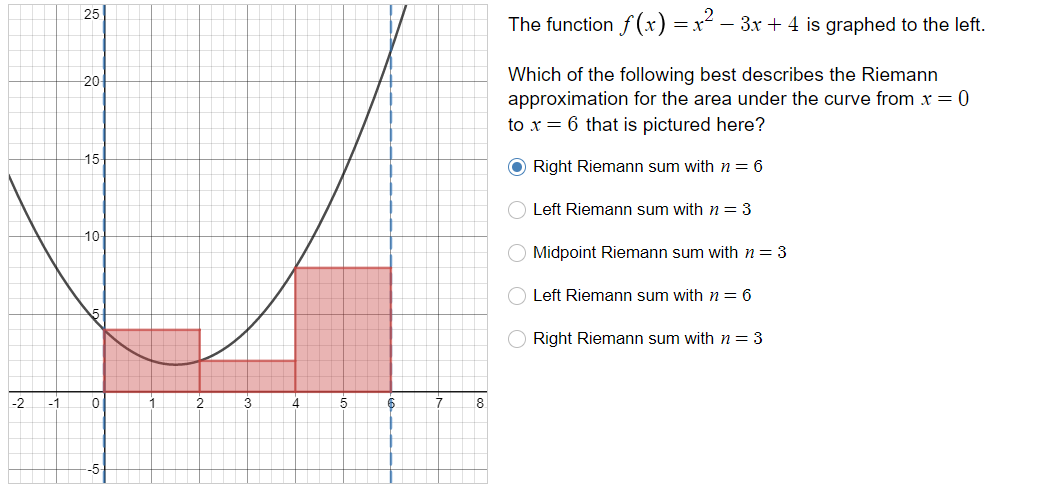# The function fx)2 -3+4 is graphed to the left25Which of the following best describes the Riemannapproximation for the area under the curve from x-0to x = 6 that is pictured here?2015Right Riemann sum with n =Left Riemann sum with n-3Midpoint Riemann sum with n = 3Left Riemann sum with n 610Right Riemann sum with n-32 -10-5

Question
3 viewshelp_outlineImage TranscriptioncloseThe function fx)2 -3+4 is graphed to the left 25 Which of the following best describes the Riemann approximation for the area under the curve from x-0 to x = 6 that is pictured here? 20 15 Right Riemann sum with n = Left Riemann sum with n-3 Midpoint Riemann sum with n = 3 Left Riemann sum with n 6 10 Right Riemann sum with n-3 2 -10 -5 fullscreen
check_circle

Step 1

To validate the correct option which is the best approximation to the area (definite integral)

Step 2

f (x) at the nodes of the partitions

Step 3

### Want to see the full answer?

See Solution

#### Want to see this answer and more?

Solutions are written by subject experts who are available 24/7. Questions are typically answered within 1 hour.*

See Solution
*Response times may vary by subject and question.
Tagged in

### Integration